# Cubic crystal system

Cubic crystal system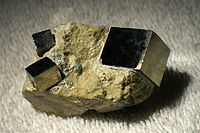A rock containing three crystals of pyrite (FeS2). The crystal structure of pyrite is simple cubic, and this is reflected in the cubic symmetry of its natural crystal facets.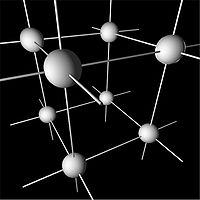A network model of a simple cubic system.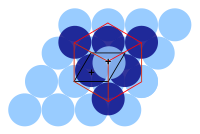The primitive and cubic close-packed (also known as face-centered cubic) unit cells.

In crystallography, the cubic (or isometric) crystal system is a crystal system where the unit cell is in the shape of a cube. This is one of the most common and simplest shapes found in crystals and minerals.

There are three main varieties of these crystals, called simple cubic (sc), body-centered cubic (bcc), and face-centered cubic (fcc, also known as cubic close-packed or ccp), plus a number of other variants listed below. Note that although the unit cell in these crystals is conventionally taken to be a cube, the primitive unit cell often is not. This is related to the fact that in most cubic crystal systems, there is more than one atom per cubic unit cell.

## Cubic space groups

The three Bravais lattices which form cubic crystal systems are:

Cubic Bravais lattices
Primitive (P) Body-centered (I) Face-centered (F)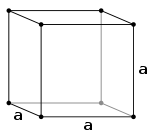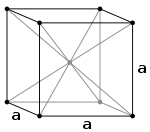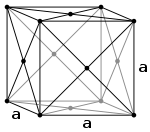The simple cubic system (P) consists of one lattice point on each corner of the cube. Each atom at a lattice point is then shared equally between eight adjacent cubes, and the unit cell therefore contains in total one atom (18 × 8).
The body-centered cubic system (I) has one lattice point in the center of the unit cell in addition to the eight corner points. It has a net total of 2 lattice points per unit cell (18 × 8 + 1).
The face-centered cubic system (F) has lattice points on the faces of the cube, that each gives exactly one half contribution, in addition to the corner lattice points, giving a total of 4 lattice points per unit cell (18 × 8 from the corners plus 12 × 6 from the faces).

Attempting to create a C-centered cubic crystal system (i.e., putting an extra lattice point in the center of each horizontal face) would result in a simple tetragonal Bravais lattice.

## Crystal classes

The isometric crystal system class names, examples, Schönflies notation, Hermann-Mauguin notation, point groups, International Tables for Crystallography space group number, orbifold, type, and space groups are listed in the table below. There are a total 36 cubic space groups.

# Point group Example Type Space groups
Class Schönflies Intl Orbifold Coxeter
195-199 Tetartoidal T 23 332 [3,3]+ Ullmannite enantiomorphic P23 F23 I23 P213 I213
200-206 Diploidal Th 2/m3
(m3)
3*2 [3+,4] Pyrite centrosymmetric Pm3 Pn3 Fm3 Fd3 I3 Pa3 Ia3
207-214 Gyroidal O 432 432 [3,4]+ Petzite enantiomorphic P432 P4232 F432 F4132 I432 P4332 P4132 I4132
215-220 Hextetrahedral Td 43m *332 [3,3] Sphalerite P43m F43m I43m P43n F43c I43d
221-230 Hexoctahedral Oh 4/m32/m
(m3m)
*432 [3,4] Galena centrosymmetric Pm3m Pn3n Pm3n Pn3m Fm3m Fm3c Fd3m Fd3c Im3m Ia3d

Other terms for hexoctahedral are: normal class, holohedral, ditesseral central class, galena type.

## Atomic packing factors and examples

One important characteristic of a crystalline structure is its atomic packing factor. This is calculated by assuming that all the atoms are identical spheres, with a radius large enough that each sphere abuts the next. The atomic packing factor is the proportion of space filled by these spheres.

Assuming one atom per lattice point, in a simple cubic lattice with cube side length a, the sphere radius would be a2 and the atomic packing factor turns out to be about 0.524 (which is quite low). Similarly, in a bcc lattice, the atomic packing factor is 0.680, and in fcc it is 0.740. The fcc value is the highest theoretically possible value for any lattice, although there are other lattices which also achieve the same value, such as hexagonal close packed and one version of tetrahedral bcc.

As a rule, since atoms in a solid attract each other, the more tightly-packed arrangements of atoms tend to be more common. (Loosely packed arrangements do occur, though, for example if the orbital hybridization demands certain bond angles.) Accordingly, the simple-cubic structure, with especially low atomic packing factor, is rare in nature, but is found in polonium. The bcc and fcc, with their higher densities, are both quite common in nature. Examples of bcc include iron, chromium, tungsten, and niobium. Examples of fcc include lead (for example in lead(II) nitrate), aluminum, copper, gold and silver.

## Multi-element compounds

Compounds that consist of more than one element (e.g. binary compounds) often have crystal structures based on a cubic crystal system. Some of the more common ones are listed here.

### Interpenetrating simple cubic (caesium chloride) structure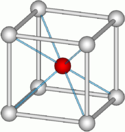A caesium chloride unit cell. The two colors of balls represent the two types of atoms.

One structure is the "interpenetrating simple cubic" structure, also called the "caesium chloride" structure. Each of the two atom types forms a separate simple cubic lattice, with an atom of one type at the center of each cube of the other type. Altogether, the arrangement of atoms is the same as body-centered cubic, but with alternating types of atoms at the different lattice sites. (See picture here.)

Examples of compounds with this structure include caesium chloride itself, as well as certain other alkali halides when prepared at low temperatures or high pressures. More generally, this structure is more likely to be formed from two elements whose ions are of roughly the same size (for example, ionic radius of Cs+ = 167 pm, and Cl = 181 pm) .

The space group of this structure is called Pm3m (in Hermann–Mauguin notation), or "221" (in the International Tables for Crystallography). The Strukturbericht designation is "B2".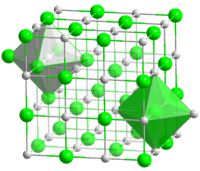The rock-salt crystal structure. Each atom has six nearest neighbors, with octahedral geometry.

The coordination number of each atom in the structure is 8: the central cation is coordinated to 8 anions on the corners of a cube as shown, and similarly, the central anion is coordinated to 8 cations on the corners of a cube.

### Rock-salt structure

Another structure is the "rock salt" or "sodium chloride" (halite) structure. In this, each of the two atom types forms a separate face-centered cubic lattice, with the two lattices interpenetrating so as to form a 3D checkerboard pattern. (See picture here.)

Examples of compounds with this structure include sodium chloride itself, along with almost all other alkali halides, and "many divalent metal oxides, sulfides, selenides, and tellurides". More generally, this structure is more likely to be formed if the cation is slightly smaller than the anion (a cation/anion radius ratio of 0.414 to 0.732).

The space group of this structure is called Fm3m (in Hermann–Mauguin notation), or "225" (in the International Tables for Crystallography). The Strukturbericht designation is "B1".

The coordination number of each atom in this structure is 6: each cation is coordinated to 6 anions at the vertices of an octahedron, and similarly, each anion is coordinated to 6 cations at the vertices of an octahedron.

The interatomic distance (distance between cation and anion, or half the unit cell length a) in some rock-salt-structure crystals are: 2.3 Å (2.3 × 10−10 m) for NaF, 2.8 Å for NaCl, and 3.2 Å for SnTe.

### Zincblende structure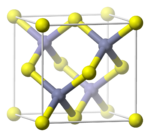A zincblende unit cell

Another common structure is the "zincblende" structure (also spelled "zinc blende"), named after the mineral zincblende (sphalerite). As in the rock-salt structure, the two atom types form two interpenetrating face-centered cubic lattices. However, it differs from rock-salt structure in how the two lattices are positioned relative to one another. The zincblende structure has tetrahedral coordination: Each atom's nearest neighbors consist of four atoms of the opposite type, positioned like the four vertices of a regular tetrahedron. Altogether, the arrangement of atoms in zincblende structure is the same as diamond cubic structure, but with alternating types of atoms at the different lattice sites. (See picture here.)

Examples of compounds with this structure include zincblende itself, many compound semiconductors (such as gallium arsenide and cadmium telluride), and a wide array of other binary compounds.

The space group of this structure is called F43m (in Hermann–Mauguin notation), or "#216" in the International Tables for Crystallography. The Strukturbericht designation is "B3".

Wikimedia Foundation. 2010.

### Look at other dictionaries:

• cubic crystal system — kubinė kristalografinė sistema statusas T sritis fizika atitikmenys: angl. cubic crystal system; cubic crystallographic system; cubic system vok. kubisches Kristallsystem, n; reguläres Kristallsystem, n; tesserales Kristallsystem, n rus.… …   Fizikos terminų žodynas

• cubic crystallographic system — kubinė kristalografinė sistema statusas T sritis fizika atitikmenys: angl. cubic crystal system; cubic crystallographic system; cubic system vok. kubisches Kristallsystem, n; reguläres Kristallsystem, n; tesserales Kristallsystem, n rus.… …   Fizikos terminų žodynas

• crystal system — n. any of the seven groups (cubic, hexagonal, rhombohedral, tetragonal, orthorhombic, monoclinic, and triclinic) of crystals classified on the basis of the relationships of their crystallographic axes (imaginary lines of reference used to… …   English World dictionary

• Crystal system — Diamond crystal structure consists of face centered cubic lattice. In crystallography, the terms crystal system, crystal family, and lattice system each refer to one of several classes of space groups, lattices, point groups, or crystals.… …   Wikipedia

• Tetragonal crystal system — In crystallography, the tetragonal crystal system is one of the 7 lattice point groups. Tetragonal crystal lattices result from stretching a cubic lattice along one of its lattice vectors, so that the cube becomes a rectangular prism with a… …   Wikipedia

• Orthorhombic crystal system — An example of the orthorhombic crystals, aragonite In crystallography, the orthorhombic crystal system is one of the seven lattice point groups. Orthorhombic lattices result from stretching a cubic lattice along two of its orthogonal pairs by two …   Wikipedia

• Monoclinic crystal system — An example of the monoclinic crystals, orthoclase In crystallography, the monoclinic crystal system is one of the 7 lattice point groups. A crystal system is described by three vectors. In the monoclinic system, the crystal is described by… …   Wikipedia

• Rhombohedral crystal system — In crystallography, the rhombohedral (or trigonal) crystal system is one of the seven lattice point groups, named after the two dimensional rhombus. A crystal system is described by three basis vectors. In the rhombohedral system, the crystal is… …   Wikipedia

• crystal system — noun each of seven categories of crystals (cubic, tetragonal, orthorhombic, trigonal, hexagonal, monoclinic, and triclinic) classified according to the possible relations of the crystal axes …   English new terms dictionary

• Cubic lattice — may refer to: Cubic crystal system Cubic honeycomb Integer lattice This disambiguation page lists articles associated with the same title. If an internal link led you here …   Wikipedia